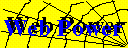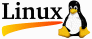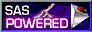# Power Analysis for ANOVA Designs

This form runs a SAS program that calculates power or sample size needed to attain a given power for one effect in a factorial ANOVA design. The program is based on specifying Effect Size in terms of the range of treatment means, and calculating the minimum power, or maximum required sample size.

### Power Form

• Number of levels (a) of effect 'A'
• Total number of levels (b) of all other factors crossed with effect 'A'.
• Error level (alpha) for which you want power or sample size calculated
• Effect size(s) for which you want power or sample size calculated
• Output: Power table Sample-size table&The calculations are performed using a SAS macro program, fpower.sas, which is somewhat more general than presented here. The web interface is written as a perl script, power.pl. This script uses the cgi-utils.pl by Lincoln Stein.